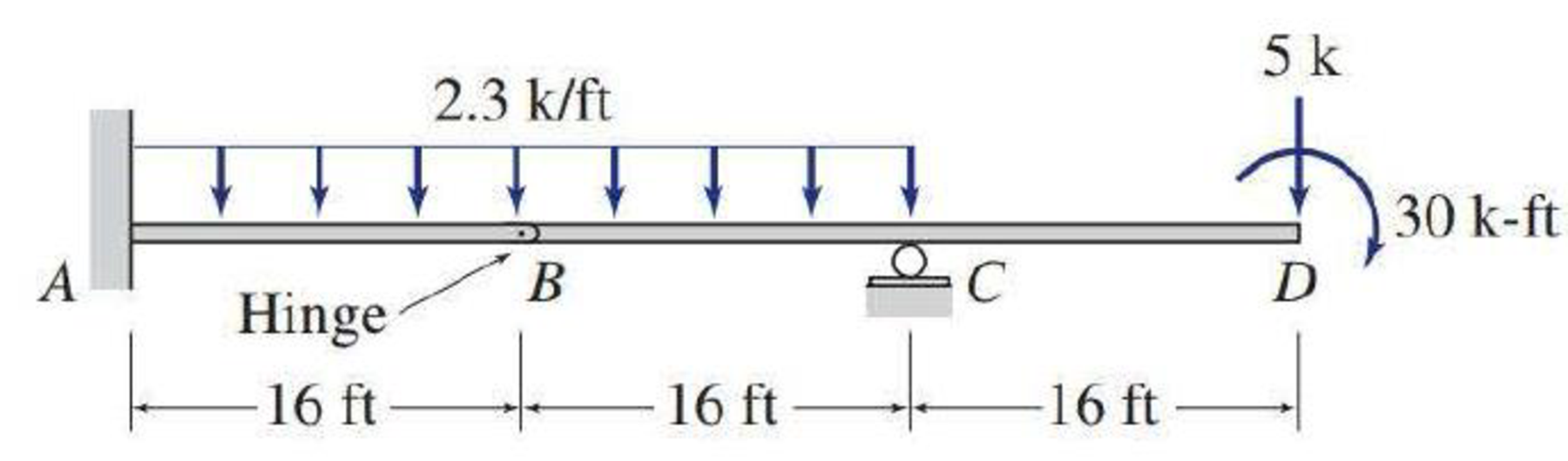# 5.29 through 5.51 Draw the shear and bending moment diagrams and the qualitative deflected shape for the beam shown.

#### Solutions

Chapter
Section
Chapter 5, Problem 46P
Textbook Problem
26 views

## 5.29 through 5.51 Draw the shear and bending moment diagrams and the qualitative deflected shape for the beam shown.To determine

Plot the shear and bending moment diagram and the qualitative deflected shape.

### Explanation of Solution

Sign conversion:

Apply the sign convention for calculating the equations of equilibrium as below.

• For the horizontal forces equilibrium condition, take the force acting towards right side as positive (+) and the force acting towards left side as negative ().
• For the vertical forces equilibrium condition, take the upward force as positive (+) and downward force as negative ().
• For moment equilibrium condition, take the clockwise moment as negative and counter clockwise moment as positive.

Apply the following sign convention for calculating the shear and bending moments.

• When the portion of the beam considered is left of the section, then the external force acting to the left are considered as positive.
• When the portion of the beam considered is right of the section, then the external force acting to the right are considered as positive.
• When the portion of the beam considered is left of the section, then the external force acting upward are considered as positive.
• When the portion of the beam considered is right of the section, then the external force acting downward are considered as positive.
• When the portion of the beam considered is left of the section, then the clockwise moments are considered as positive.
• When the portion of the beam considered is right of the section, then the counterclockwise moments are considered as positive.

Calculation:

Show the free-body diagram of the entire beam as in Figure 1.

Consider the section BCD.

Find the vertical reaction at point C by taking moment about the point B.

+MBBCD=02.3(16)(162)305(32)+Cy(16)=0294.430160+16Cy=0Cy=30.275k

Find the horizontal reaction at point A by resolving the horizontal equilibrium.

+Fx=0Ax=0Ax=0

Find the moment at point A by taking moment about point A.

+MA=0MA2.3(32)(322)+30.275(32)5(48)30=0MA1177.6+968.824030=0MA=478.8k-ft

Find the vertical reaction at point A by resolving the vertical equilibrium.

+Fy=0Ay2.3(32)+Cy5=0Ay73.6+30.2755=0Ay=48.325k

Show the reactions of the beam as in Figure 2.

Shear diagram:

Point A:

SA,L=0SA,R=48.325k

Point B:

SB=48.3252.3(16)=11

### Still sussing out bartleby?

Check out a sample textbook solution.

See a sample solution

#### The Solution to Your Study Problems

Bartleby provides explanations to thousands of textbook problems written by our experts, many with advanced degrees!

Get Started

Find more solutions based on key concepts
The masonry wall shown in Figure 13.27 is commonly referred to as a Trombe wall in honor of Felix Trombe, who d...

Engineering Fundamentals: An Introduction to Engineering (MindTap Course List)

Explain how resolution affects printer quality.

Enhanced Discovering Computers 2017 (Shelly Cashman Series) (MindTap Course List)

What type of metals should not be ground on a pedestal grinder?

Precision Machining Technology (MindTap Course List)

Write the query that will show only the duplicate customer records.

Database Systems: Design, Implementation, & Management

List the sections of a system design specification, and describe the contents.

Systems Analysis and Design (Shelly Cashman Series) (MindTap Course List)

What is the difference between DAO and RDO?

Database Systems: Design, Implementation, & Management

Why are arc strikes outside the welding zone considered a problem on pipe?

Welding: Principles and Applications (MindTap Course List)

If your motherboard supports ECC DDR3 memory, can you substitute non-ECC DDR3 memory?

A+ Guide to Hardware (Standalone Book) (MindTap Course List)